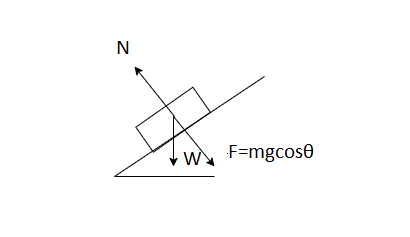Normal Force homework Help at TutorEye

### How to find normal force on an incline?

When an object is placed on the inclined plane, its weight is resolved into normal force. This force is equal to perpendicular to plane.Normal force equals to Fcosθ

N =W = Fcosθ

### How to find the magnitude of normal force?

Normal force can be calculated by finding the weight of the object.

Weight = mg
Where m is mass of object
g is acceleration due to gravity
N = mgcosθ
This is the magnitude of normal force.